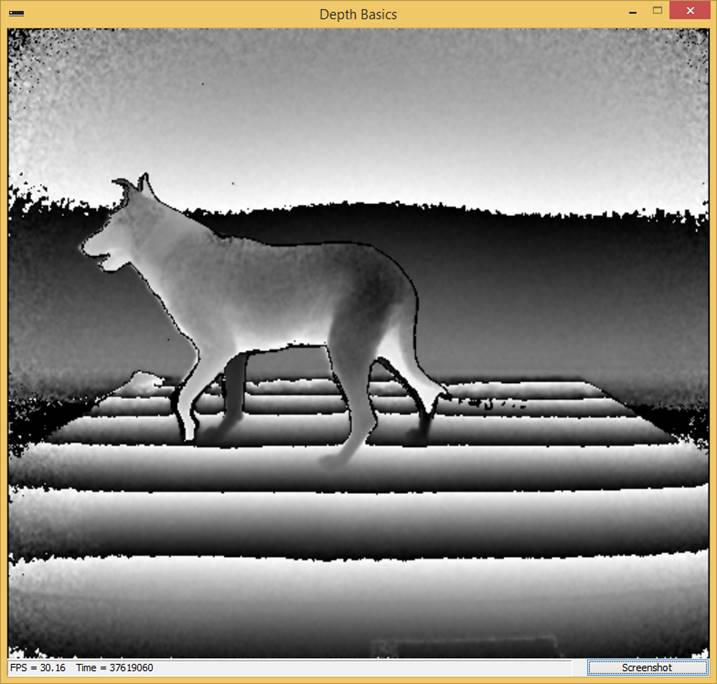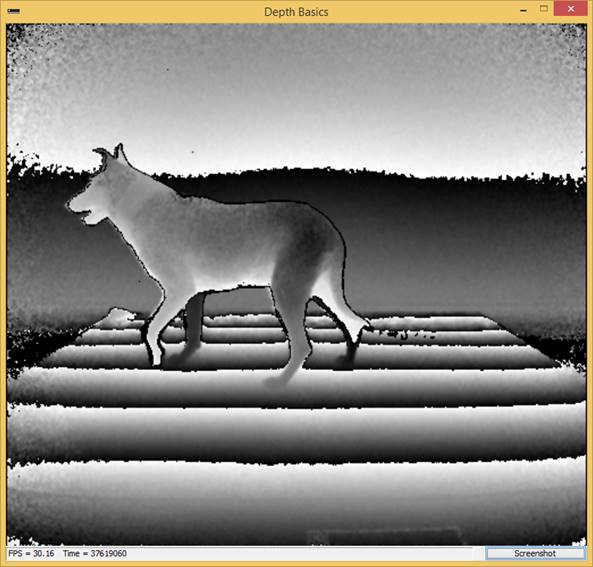# Background and floor removal from depth camera dataPetro Soininen

Computer vision is used in various industries to process camera data as part of an analytics pipeline. Examples of usage of computer vision range from automated surveillance camera systems in public spaces to quality and stability monitoring of paper machines.

This document introduces a reusable pattern and set of algorithms for extracting objects of interest from depth camera data.

Commodity depth-capable cameras, such as the Microsoft Kinect, provide a wealth of cost-efficient opportunities for detection and tracking of various shapes and objects. One interesting research area for utilizing depth camera data revolves around a combination of machine learning and model fitting to identify and track hand movement with extremely high fidelity in real time. A video about how Microsoft Research approaches this scenario can be found here.

We have had several discussions with academia and enterprise partners about their ideas and needs for object detection – especially moving objects such as animals – and how to utilize a combination of commodity hardware and software to detect poses and movement of these objects.

The Microsoft Research assets for hand tracking provided a useful baseline for us to prototype our ideas for implementing non-human movement and pose tracking. In this case study, we share a generalizable solution for something almost all developers working with depth camera data need to tackle: the need to separate a specific object of interest from the rest of the image.

## Overview of the Solution

As an example scenario, we recorded depth data of a space with Ilkka’s dog Mici posing majestically for us. Below is a sample raw depth frame from the Kinect sensor – let’s take a look at how to strip out the uninteresting background from the data.Initially, our assumption was that it would be enough to separate objects of interest by removing the redundant background by implementing floor removal functionality with Kinect SDK API and setting the background clipping distance to also remove the walls and other rigid parts of the observed space. Although initial results with this approach were promising, those clipping plane settings wouldn’t work with all of our Kinect depth recording reference data. Therefore, we had to design a universal solution that wouldn’t be affected by variations in the setup.

Pseudo-code for the key parts of the algorithm to extract a region of interest from depth data are included below, with more technical details and C++ implementations available in the kinect-bits code repository.

### Floor removal

The code below describes the idea behind calculating the camera space position and the distance to the floor for depth data pixels. Point-plane distance from the pixel to the calculated floor plane can then be observed to determine whether the pixel is a part of the floor to be removed.

```// Floor removal

Set cameraSpacePoints to CameraSpacePointArray[DepthImageWidth*DepthImageHeight];
Set floorClipPlane to Kinect API floor clip plane;
Set threshold to 0.02;
If FloorClipPlaneDetected is TRUE

// Convert depth pixel values to camera space points
MapDepthFrameToCameraSpace(depthImage, cameraSpacePoints);

// Iterate all points in camera space points array
Foreach(spacePoint in cameraSpacePoints)
{
// Calculate Point-Plane Distance from floor plane to camera space point
distance = PointPlaneDistance(spacePoint, floorClipPlane);
If distance is less than threshold
Set pixel value at corresponding index on depth frame to zero;
}
```

The screenshots below illustrate our reference depth data before and after applying floor removal.### Background removal and Region of Interest estimation

Now that the floor plane has been stripped out, let’s focus on retaining just the core region of interest.

Because a depth image frame consists of an array of integer values, we can simply apply arithmetic operations on it. If we subtract the depth image containing only the background of the setting (e.g. room) from another depth image that includes the object of interest, the result will be an image with only the differing pixels, the object of interest. In addition, due to noise on the result frame, we apply a filter to exclude the stray pixels that aren’t part of the object.

#### Background removal

In order to produce a more precise understanding of the setting background and the object we want to extract, our algorithm does the following:

• looks at sequential depth frames from the reference setting without the object of interest being present,
• prepares the reference data by applying the average value of the swept row to pixels that might have zero values due to anomalies in the captured, raw depth data.
``````/* Replace invalid pixels in depth images (pixels with 0 value)
with pixel average value from stride */

For (i = 0; i < collectedImageCount; i++)
{
For (y = 0; y < imageHeight; y++)
{
Set zero_fill to 0;
Set count to 0;

// Calculate pixel average from stride
For (p = 0; p < imageWidth; p++)
{
Set value to pixel value in depth image at index [p + (y * imageWidth)];

If value is not 0
Increase count by 1;
}

If count is not 0
Divide zero_fill by count;

// Fill zero pixels in image with calculated average pixel value
For (x = 0; x < imageWidth; x++)
{
Get pixel value in depth image at index [x + (y * nWidth)];
If pixel value is not zero
Keep pixel value in depth image;
Else
Set pixel in image to calculated average pixel value;
}
}
}
``````
• Subtracts the average of the reference data from the depth frames that include the object of interest
• if the difference between particular pixels is greater than a threshold value (dependent on, for example, the distance to the object, or the amount of noise on the frame) – the pixel is considered to belong to a foreground object
``````/* Calculate average of the pixels
to get the reference depth image to be used background removal */

For (i = 0; i < imageSize; i++)
Set pixel value at index i in depth image to pixel value divided by 10;

// Calculate difference of input depth image and reference depth image
For (i = 0; i < size; i++)
{
Set depth_value to pixel value in input depth image at index i;

If depth_value is 0
Set depth_value to pixel value in reference depth image at index i;

Subtract depth_value from pixel value in reference depth image at i;

If difference is greater than 200
Store reference value into result image;
Else
Store background value (5000) into result image;
}
``````

#### Region of Interest Estimation

Once the foreground pixels have been identified, object boundaries can be estimated by looking at the coordinates of the foreground pixel map.

To help make sure the estimated region is not impacted by potential stray pixels caused by the object entering the scene, the algorithm uses an 8×8 pixel kernel as a viewport to help determine whether that specific area of the frame is on top of the foreground object.

``````// Calculate 2d coordinates for each kernel in-depth image

Set kernel_index to 0;
Set kernel_index_start_y to 0;
Set min_x to DepthImageWidth;
Set min_y to DepthImageHeight;
Set max_x to 0;
Set max_y to 0;
Set kernel_row to 0;
Set kernel_size to 8;
Set HorizontalBlocks to DepthImageWidth / KernelSize;
Set VerticalBlocks to DepthImageHeight / KernelSize;

For (y = 0; y < DepthImageHeight; y++)
{
For (x = 0; x < DepthImageWidth; x++)
{
Set pixel_value to value in depth image at [(y * DepthImageWidth) + x];

If pixel_value is 5000
Increase background pixel count in kernel in kernel array at kernel_index;

If modulo of x and kernel_size is 0 AND x is not 0
Increase kernel_index by 1;

// Last pixel was handled on current row
If x is DepthImageWidth - 1
// Last horizontal kernel handled
If modulo of y and kernel_size is 0 AND y is not 0
// Calculate 2d coordinates for each kernel on that row (horizontally)
for (kernel = 0; kernel < HorizontalBlocks; kernel++)
{
Calculate top left x coordinate
into kernel array at [kernelIndexStartY + kernel]
by multiplying kernel index with kernel_size;
Calculate top left y coordinate
into kernel array at [kernelIndexStartY + kernel]
by multiplying kernel_row with kernel_size;
}

Increase kernel_index_start_y by HorizontalBlocks
to update the index to next kernel row in kernel array
Increase kernel_row by one to handle next horizontal row
Set kernel_index to kernel_index_start_y to access next kernel
}
}
``````

Object boundaries can then be calculated by using the coordinates from pixel kernels determined to belong to the foreground object – i.e., from the kernels in which the amount of background data pixels is not above a threshold value.

``````// Calculate bounding box of the object using kernels pixel counts

Set BackgroundPixelCountThreshold to 16
For (kernel = 0; kernel < HorizontalBlocks*VerticalBlocks; kernel++)
{
If pixel count in kernel array at kernel index
is smaller than BackgroundPixelCountThreshold

If top left x coordinate is smaller than min_x
Set min_x to top left x coordinate in kernel array at kernel index;
If top left x coordinate is greater than max_x
Set max_x to top left x coordinate in kernel array at kernel index;
If top left y coordinate is smaller than min_y
Set min_y to top left y coordinate in kernel array at kernel index;
If top left y coordinate is greater than max_y
Set max_y to top left y coordinate in kernel array at kernel index;
}
``````

With the foreground object boundaries calculated, the final step is to extract the region – and in our example app, draw it to the screen with a bounding box. Below are example screenshots of our extracted region of interest, starting point, and the intermediate step, after applying floor removal.Extracted region of interest